# Compensating Current Feedback Amplifiers in Photocurrent Applications

### Introduction

Historically, current feedback amplifiers (CFA) have not been the first choice for use as transimpedance amplifiers (TIA) due to their relatively high inverting input currents and inverting input current noise, which can be at least an order of magnitude larger than that of a comparable voltage feedback amplifier (VFA). Additionally, many system designers are unfamiliar with CFAs, so they’re less comfortable using them. The fact remains, however, that CFAs are quite easy to use and can outperform their VFA counterparts in applications that require high gain, low power, low noise, wide bandwidth, and high slew rate. One of their main benefits is that the loop gain of an ideal CFA is independent of its closed-loop gain, thus allowing the CFA to deliver excellent harmonic distortion and bandwidth performance irrespective of its closed-loop gain.

Due to their very low input bias current and input current noise, FET-input op amps are often given the highest consideration for TIA applications, particularly those that use low output current devices, such as photoelectric elements, as the input current source. While FET-input amplifiers do excel in many of these applications, their speed can be insufficient in systems that require faster performance. Thus, CFAs are increasingly being used as TIAs in faster systems that can tolerate more noise.

This article deals with how the parasitic capacitance of a photodiode or other light-to-current transducer affects a CFA operating as a TIA, and how to properly compensate the amplifier for this capacitance. Some introductory material regarding CFA operation is provided, as well as occasional parallels between CFA and VFA analyses. Analysis of the “noise gain” of VFA circuits or “feedback impedance” of CFA circuits is not used. Instead, classical feedback theory using loop gain is used to avoid difficulties incurred when moving between current and voltage domains (loop gain is always a dimensionless quantity) and because the theory itself presents Bode plots that are straightforward and easy to use.

### Current Feedback Amplifier Basics

An ideal CFA has zero input impedance—a dead short across its inputs—because the negative feedback signal is a current. In contrast, an ideal VFA has infinite input impedance because its feedback signal is a voltage. The CFA senses the error current flowing in its input and develops an output voltage equal to Z times the input current, where Z represents the transimpedance gain. The direction of the error current is defined to produce negative feedback. Similar to A in a VFA, Z approaches infinity in an ideal CFA. Figure 1 shows the basics of how an ideal CFA could be configured as a TIA to transfer the current from an ideal current source to its output voltage.

The closed-loop gain of this TIA can be expressed as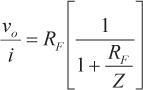(1)

Equation 1 shows that as Z approaches infinity, the TIA gain approaches its ideal value of RF. As Z approaches infinity, the error current, ie, approaches zero, and all of the input current flows through RF. The loop gain is seen as

in Equation 1.

Unfortunately, ideal CFAs do not exist, so practical devices use the next best thing: a unity-gain buffer across their inputs. A current mirror reflects the error current to a high-impedance node where it is converted to a voltage, buffered, and fed to the output, as shown in Figure 2.Figure 2. Practical CFA with unity-gain buffer used as a TIA.

As long as Ro = 0, the closed-loop gain is the same as that given in Equation 1. When Ro > 0, the closed-loop gain becomes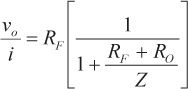(2)

and the loop gain is

### TIA Design Using Practical Components

Photodiode and other photoelectric devices exhibit a parasitic shunt capacitance proportional to the device area. When Ro = 0, this capacitance is fully bootstrapped, so it has no effect on the closed-loop response. In a real CFA, Ro > 0, and the parasitic capacitance influences the response, potentially causing the circuit to become unstable. In addition, like the open-loop gain, A, in a VFA, the magnitude of Z in a real CFA is large at low frequency and rolls off with increasing frequency, and the phase shift lags more with increasing frequency. To first order, Z(s) can be characterized with a single dominant pole at s = p and dc transimpedance of Zo, as shown in Equation 3. High frequency poles in Z(s) will be considered later.(3)

The circuit in Figure 3 includes the parasitic capacitance, C, and the transimpedance, Z(s). Note that the CFA’s inverting input capacitance can be absorbed into C.

Equation 4 is derived by performing KCL at the inverting input.(4)

The error current, ie, is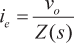(5)

Combining Equation 4 and Equation 5 produces the following result for the closed-loop TIA gain of the circuit in Figure 3:(6)

The loop gain is evident in Equation 6 and is given by(7)

The loop gain contains two poles, a low-frequency pole at s = p and a high-frequency pole at

When Ro<< RF, the parallel combination of RF and Ro can be approximated by Ro. The two poles present a stability problem when the high-frequency pole occurs at a frequency where the magnitude of the loop gain is greater than 0 dB. When Ro and C are small, the parasitic pole occurs at a frequency higher than the crossover frequency, and the amplifier is stable. This is generally not the case in most TIA circuits, however, so we must find a way to compensate for the inverting input parasitic capacitance.

### Adding a Feedback Capacitor (a Brief Digression)

A CFA with a single-pole transfer function, as given in Equation 3, is stable with any value of feedback resistor because the lagging phase shift around its feedback loop is limited to –90°. The secondary poles of real CFAs will introduce significant phase lag at high frequencies, however, which places a practical limit on the minimum value of RF to ensure stability (45° is often the minimum acceptable phase margin). From here on, Z(s) will include a high-frequency pole at s = pH, along with the dominant pole s = p.

To ensure that the feedback impedance does not go to zero, common advice says that we shouldn’t use a feedback capacitor in any CFA circuit. It’s not that simple, however, since the feedback capacitor introduces phase shift, in addition to magnitude changes. This section looks at what happens when a feedback capacitor is added to a CFA-based TIA, omitting the parasitic input capacitance for the moment. Adding a feedback capacitor, CF, across the feedback resistor, RF, in the circuit shown in Figure 2 produces a pole and a zero in the loop gain. ZF is defined as the parallel combination of RF and CF: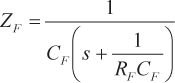(8)

If RF in Equation 2 is replaced with ZF, then the closed-loop gain is as expressed in Equation 9.(9)

The loop gain is then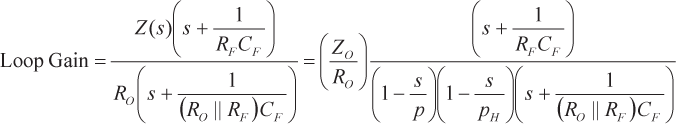(10)

The loop gain has a dominant pole at s = p and a high-frequency pole at s = pH from Z(s). In addition, it has a pole at

and a zero at

due to the added feedback capacitor.

In the Bode plot, the zero due to CF occurs at a lower frequency than the pole due to CF because the zero frequency expression contains RF in the denominator, and the pole frequency expression contains (Ro||RF) in the denominator. The Bode plot for one possible CFA-based TIA with CF (Equation 10) is shown in Figure 4.

The zero produces increasing magnitude and leading phase shift with increasing frequency, which can, in some situations, be a good thing from a stability standpoint. In the system modeled in Figure 4, however, the zero pushes out the point where the loop gain crosses 0 dB, and the pole at pH causes the magnitude asymptote to drop at –40 dB/decade beyond crossover. The dashed blue line shows the loop gain without CF, using Equation 2 and the two-pole version of Z(s), as expressed in Equation 11.(11)

Figure 4 shows that the amplifier is stable without CF but develops stability problems when CF is added. The plot in Figure 4 does not completely preclude the use of a feedback capacitor, as this particular Z(s) is not representative of all CFAs and actual resistor and capacitor values are not used, but it does show that the high-frequency pole limits how much feedback capacitance can be safely applied. Figure 4 also shows that any amount of feedback capacitance could be safely added to a hypothetical CFA with a single-pole transfer function and that adding the feedback capacitance would extend its closed-loop bandwidth.

### Using the Zero Due to CF to Cancel the Pole Due to the Parasitic Capacitance

Now that the effect of adding CF to a CFA is understood in a general sense, it can be shown that CF can be safely used to compensate for the parasitic shunt capacitance of an input current source.

The closed-loop gain of the circuit in Figure 3 is indicated in Equation 6. In order to see what happens to this circuit when a feedback capacitor is added, RF can be replaced by ZF in Equation 6, similar to what was done to develop Equation 9, where ZF is defined in Equation 8. The circuit is shown in Figure 5.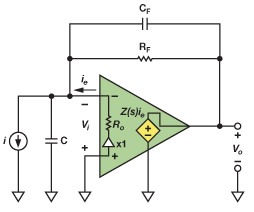Figure 5. Practical CFA-based TIA with CF used to compensate parasitic capacitance.

The closed-loop gain of the circuit in Figure 5 is given in Equation 12(12)

from which the loop gain can be determined to be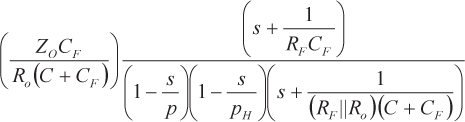(13)

The zero due to CF in Equation 13 is the same as the zero in Equation 10, but the pole due to CF has moved from

to

The addition of C to CF allows the pole position to be moved to match the zero position, thus canceling out the pole due to the parasitic capacitance, C, of the input current source. Setting the pole frequency due to CF and C equal to the zero frequency due to CF in Equation 13 yields Equation 14:(14)

Equation 14 shows the simple formula to calculate the value of CF, which cancels the pole in the loop gain due to the parasitic capacitance, C, in the TIA shown in Figure 5. With this perfect pole-zero cancellation, the loop gain reverts back to its original form with dominant and high-frequency poles as in Equation 11. The closed-loop gain can now be expressed as shown in Equation 15.(15)

The main difficulty encountered when using Equation 14 is determining Ro, which can be variable, and is not always specified in CFA data sheets. The pole-zero cancellation does not need to be exact, however, as long as the slope of the loop gain plot is reasonably close to –20 dB/decade as it passes through 0 dB. Equation 14 shows that CF decreases linearly with Ro due to the increasing bootstrapping that occurs as Ro approaches 0, where C becomes fully bootstrapped and the required CF equals 0. Equation 14 can also be expressed in a matched time constant form as RoC = RFCF. The matched time constant form of Equation 14 bears a strong resemblance to the result obtained when compensating VFAs for parasitic summing node capacitance: RGCG = RFCF, where RG is the VFA gain resistor and CG is the capacitance across RG, which is usually the parasitic summing-node capacitance. There is, however, a price to pay for this benefit. While adding CF stabilizes the TIA, it also introduces a pole in the closed-loop gain at

as can be seen in Equation 12 and Equation 15. The closed-loop gain described by Equation 15 can be thought of as two cascaded systems with their transfer functions multiplied together. The first system has the leftmost factor in Equation 15 as its transfer function and has dimensions of ohms. The second has the rightmost factor in Equation 15 as its transfer function and is dimensionless.

The response of the second system is governed by the loop gain and can be modeled by a first-order transfer function as long as the loop gain magnitude crosses 0 dB at –20 dB/decade. Basic feedback theory shows that if this roll-off condition is met, the closed-loop gain magnitude of the second system is approximately unity when the loop gain magnitude is >>1, and follows the loop gain magnitude when the loop gain magnitude is <<1. The 3-dB point in the closed-loop gain occurs at the frequency where the loop gain magnitude crosses 0 dB(if the slope is a little faster than –20 dB/decade, some peaking will occur in the closed-loop response near the 0-dB crossover point). In a stable amplifier, the second system can, therefore, be approximated as a first-order, low-pass filter with unity gain in the pass-band and cutoff frequency equal to the frequency, where the loop gain magnitude crosses 0 dB. The transfer function of the first system is the reciprocal of the feedback factor and has a simple first-order, low-pass response with a dc value of RF and corner frequency of

Intuitively, the additional pole due to CF makes sense because the output voltage is developed by current flowing through the feedback impedance, which decreases with increasing frequency. The pole forms where the reactance of CF is equal to the value of RF. This same situation occurs in VFA-based TIAs that use feedback capacitor compensation. The closed-loop bandwidth can, however, be broadened somewhat by cautiously decreasing CF from the value calculated in Equation 14, moving the pole frequency out, and reducing phase margin, but this must be done experimentally.

### Simulation Data

To test this result, a simple simulation model for a CFA was developed with Zo = 1 MΩ, p = –2π (100 kHz), pH = –2π (200 MHz), Ro = 50 Ω, and RF = 500 Ω. The magnitude of the loop gain is found by taking the magnitude of Equation 11 with these values.(16)

which equals 1 at approximately f = 145 MHz.

The loop gain phase shift at 145 MHz is given(17)

resulting in approximately 54° of phase margin, which is a reasonable place to start for a basic CFA with no parasitic capacitances.

Figure 6 shows the simulation of the response of this model to a 1-ns rise time current step input.Figure 6. Basic TIA step response with no parasitic capacitance (20 ns/div).

The response is clean, with minimal ringing—just what would be expected with 54° of phase margin. The step response of the same amplifier with 50 pF of parasitic capacitance added between the inverting input and ground is shown in Figure 7.Figure 7. Step response with 50 pF of capacitance between inverting input and ground (20 ns/div).

The vertical scale in Figure 7 is the same as it is in Figure 6, but the trace was moved down one division to accommodate the ringing. The excessive ringing is clear, and this amplifier clearly has a phase margin problem.

The amplifier can be stabilized by adding a feedback capacitor determined by Equation 14, which is calculated to be 5 pF. Figure 8 shows the results when the 5-pF feedback capacitor is added.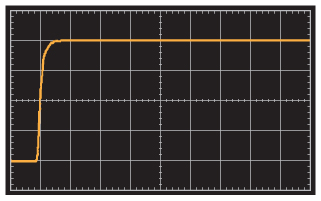Figure 8. Step response with pole/zero cancellation using 5-pF feedback capacitance (20 ns/div).

The bandlimiting due to the pole in the closed-loop gain is evident. The loop gain 0-dB crossover for the original amplifier was determined to be 145 MHz, which corresponds to a time constant of approximately 1.1 ns in a first-order system, and the RFCF time constant is 2.5 ns (note that the loop gain magnitude roll-off rate is a little faster than –20 dB/decade at the 0-dB crossover since the phase margin is less than 90°, but the first-order, closed-loop model is a reasonably accurate approximation). Using the model of two cascaded systems as described above, the aggregate time constant of the cascaded systems can be estimated to be the root-sum-square of the two time constants (the input current source 10% to 90% rise time of 1 ns corresponds to an effective sub-ns time constant that is short enough to ignore), or approximately 2.7 ns, which looks about right for the response shown in Figure 7.

Reducing CF to 3 pF reduces the phase margin somewhat and increases the closed-loop pole frequency, speeding things up as shown in Figure 9.

It’s clear that some experimentation may be necessary to get the best value for CF. Other factors such as load capacitance, board layout, and variations in Ro also factor into the selection of CF.

### Conclusion

With the increasing interest in the use of CFAs as TIAs, it is important to understand how to compensate for transducer capacitance on a CFA’s inverting input and why the compensation works. This article uses classical feedback techniques to develop a simple scheme that adds a single feedback capacitor in parallel with the feedback resistor to compensate for the inverting input capacitance. The feedback capacitor introduces an undesired pole in the closed-loop response, but the capacitor’s value can be empirically adjusted from the calculated value to reduce the pole’s band-limiting effect.

I invite you to comment on Current Feedback Amplifiers in the Analog Dialogue Community on EngineerZone.

### References

Gray, Paul R., and Robert G. Meyer. Analysis and Design of Analog Integrated Circuits. John Wiley & Sons, Inc., 1977.

Lundberg, Kent. “Feedback Control Systems.” M.I.T. Course Notes.

Roberge, James K. Operational Amplifier: Theory and Practice. John Wiley & Sons, 1975.